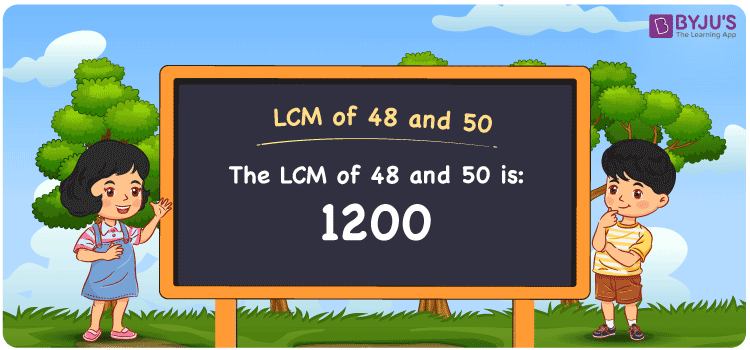# LCM of 48 and 50

LCM of 48 and 50 is 1200. The number divisible by 48 and 50 is the LCM. Least common multiple of 48 and 50 is the smallest value we get from the common multiples. (48, 96, 144, 192, 240, 288, 336, ….) and (50, 100, 150, 200, 250, 300,….) are the few multiples of 48 and 50. The LCM of 48 and 50 can be found easily using the prime factorisation method, division method and by listing the multiples.

Also read: Least common multiple

## What is LCM of 48 and 50?

The answer to this question is 1200. The LCM of 48 and 50 using various methods is shown in this article for your reference. The LCM of two non-zero integers, 48 and 50, is the smallest positive integer 1200 which is divisible by both 48 and 50 with no remainder.## How to Find LCM of 48 and 50?

LCM of 48 and 50 can be found using three methods:

• Prime Factorisation
• Division method
• Listing the multiples

### LCM of 48 and 50 Using Prime Factorisation Method

The prime factorisation of 48 and 50, respectively, is given by:

48 = 2 x 2 x 2 x 2 x 3 = 2⁴x 3¹

50 = 2 x 5 x 5 = 2¹ x 5²

LCM (48, 50) = 1200

### LCM of 48 and 50 Using Division Method

We’ll divide the numbers (48, 50) by their prime factors to get the LCM of 48 and 50 using the division method (preferably common). The LCM of 48 and 50 is calculated by multiplying these divisors.

 2 48 50 2 24 25 2 12 25 2 6 25 3 3 25 5 1 25 5 1 5 x 1 1

No further division can be done.

Hence, LCM (48, 50) = 1200

### LCM of 48 and 50 Using Listing the Multiples

To calculate the LCM of 48 and 50 by listing out the common multiples, list the multiples as shown below

Multiples of 48 = 48, 96, 144, 192, 240, 288, 336, 384, 432, 480, . . . ., 1056, 1104, 1152, 1200, . . .

Multiples of 50 = 50, 100, 150, 200, 250, 300, 350, 400, 450, 500, . . . ., 1000, 1050, 1100, 1150, 1200, . . . .

The smallest common multiple of 48 and 50 is 1200.

Therefore LCM (48, 50) = 1200

## Video Lesson on Applications of LCM## LCM of 48 and 50 Solved Examples

Question: The LCM and GCD of two numbers are 1200 and 2. If one number is 50, determine the other number.

Solution:

Consider m as the other number

GCD x LCM = 50 x m

m = (GCD x LCM)/50

m = (2 x 1200)/50

m = 48

Hence, the other number is 48.

## Frequently Asked Questions on LCM of 48 and 50

### How can we find the LCM of 48 and 50?

We can find the multiples of 48 and 50 using the common multiples. LCM is the smallest multiple divisible by 48 and 50 exactly.

### What are the methods used to get the LCM value of 48 and 50?

The methods used to get the LCM value of 48 and 50 are Prime Factorization Method, Division Method and Listing multiples.

### Calculate the GCF if the LCM of 48 and 50 is 1200.

LCM x GCF = 48 x 50

Given

LCM of 48 and 50 = 1200

1200 x GCF = 2400

GCF = 2400/1200 = 2

### What is the LCM of 48 and 50?

The LCM of 48 and 50 is 1200.

### Calculate the LCM of 48 and 50 from the numbers 1200, 16, 20, 27.

The LCM value is the smallest common multiple divisible evenly by the two given numbers. The number 1200 satisfies this condition.